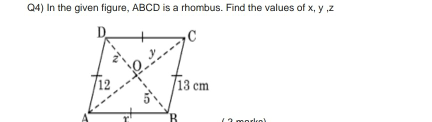"
">

# Find the values of x, y, z."

Given :

In a rhombus ABCD, $AO = 12$ $BO = 5 cm$ $BC = 13$.

To do :

We have to find the values of x and y.

Solution :

A Rhombus is a quadrilateral in which all the sides are equal.

In the given question,

$AO = 12$ $BO = 5 cm$ $BC = 13$.

In the Rhombus diagonals bisect each other.

Therefore, $AO=OC, BO=OD$

$OD=z=5$ and $OC=y=12$.

Since all sides are equal in rhombus, $AB=BC$

$AB = x=13$.

Therefore, the values of x, y, z are 13, 12, 5 respectively.

Updated on: 10-Oct-2022

46 Views